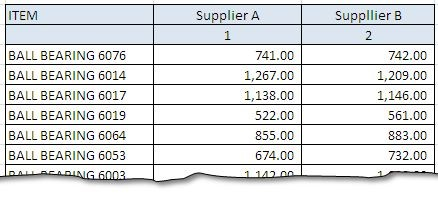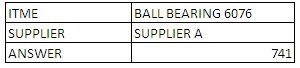# Retriving Quotation Rates For Two Parties With ExcelWhen using Excel for Procurement, it is common to use it for making quotations. When there is more then one supplier, you might come across situation when you have to retrieve the cost of a part from a specific supplier. This could be in context of a particular part, for a particular department, for a particular month and many more details could be attached to such a lookup process.

In this post, we will be discussing how to retrieve the results from a quotation or a databank containing supplier and theirs quotations for a certain part. For simplicity we will consider just two suppliers, against which we will be retrieving quoted prices.

We will take as example, the following data that lists two types of bearings – ball and roller for two suppliers – Supplier A and Supplier B. The respective prices are given below each supplier.Workout No. 01 : The VLOOKUP with MATCH combination:

The problem can be solved if we use VLOOKUP for lookup in the left most column and for MATCH to match suppliers. The final formula should like the below one:The formula gives the following result when inserted into the Excel SheetWorkout No. 02 : The VLOOKUP with HLOOKUP combination:

HLOOKUP() is another family member of lookup family. Unlike VLOOKUP, it is used to retrieve data when it is located in a row (as against column) and return values from a specified row. The function has following syntax:

HLOOKUP(lookup_value, table_array, row_index_num, [range_lookup])

The row index argument is used to define the row from where results will be retrieved.

For our example, the formula that will work for this case is mentioned below and is also elaborated.Both alternatives are equally effective and retrieve same results. The down side of the second alternative i.e. using HLOOKUP is that you have to add an additional row to list Supplier Count i.e. 1,2,.. and so on.

This was a comparatively small data that we just took up to elaborate the formula, but this simple formula can be really effective in retrieving data from large databases. Hope you have liked this post, we will revert with something new in our next post.